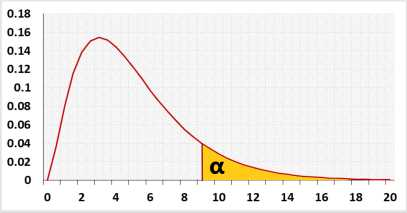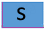# Chi-squared test for variance calculator

Unknown standard deviation
Enter raw data directly
Enter raw data from excel
Enter summarized data (n, S)

## Enter sample data

Header: You may change groups' name to the real names.
Data: When entering data, press comma , , Space or Enter after each value.

The tool will not count empty cells or non-numeric cells.

## Enter sample data

You may copy data from Excel, Google sheets or any tool that separate data with Tab and Line Feed.
Copy the data, one block of 2 consecutive columns includes the header, and paste below.

Copy the data,

It is okay to leave empty cells, empty cells or non numeric cells won't be counted

When entering raw data, the tool will run the Shapiro-Wilk normality test, calculate outliers and generate R code, as part of the test calculation.
validation message

## Information

Target: To check if the assumed variance ()σ20) is statistically correct, based on a sample variance S2.

the default is right tailed test, usually you worried only if the standard deviation is bigger then the expected one.

1. Right tailed example:
A factory uses machine to create screws. the standard deviation of the screws diameter must not exceed 0.1 mm.

2. Two tailed test example:
A factory uses a machine to create screws. recently the engineers upgraded a big module in the machine.
The quality assurance are interested to know if there was a change in the standard deviation of the screws diameter.
If the standard deviation is bigger the engineer must fix it to meet the expected quality.
if the standard deviation is smaller, sales may consider to sell screws with better standard, and higher price. Example1: A farmer calculated last year the variance of the apples' weight in his apple orchard σ20 equals 2 kg2, based on big sample
Current year he checked a small sample of apples and the sample variance S2 equals 3 kg2
Was the variance of the apple's weight changed this year?
The farmer will run the two-tailed test.

The farmer consulted with a statistician and told him that he only worry if the variance will be greater, smaller variance is not a concern.
The statistician recommend him to run the right-tailed test and check the H1: &σ2>2kg

Hypotheses
H0: σ2 = σ20
H1: σ2 < > σ20
Test statisticχ² distribution## AssumptionsNormal distributionIndependent observationsThe population expected variance is known

## Required Sample DataSample standard deviationSample size

## R Code

The following R code should produce the same results:

The R code doesn't exclude outliers.

Examples
1. Two-tailed test
A farmer calculated last year the average of the apples' weight in his apple orchard μ0 equals 17 kg, based on the entire population.
The current year he checked a small sample of apples and the sample average x̄ equals 18 kg
Has the average of the apple's weight changed this year?
The farmer calculates the sample standard deviation of the apple's weight.

2. Left-tailed test.
In the same example as above, the farmer only cares to know if the entire average is lesser this year.